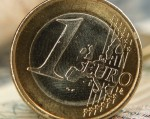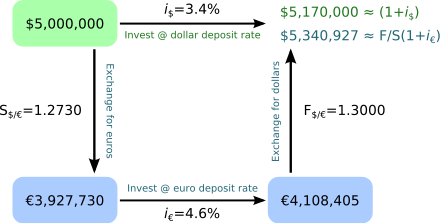# Covered Interest Arbitrage StrategyGetting a currency forward can be a tricky business even for a major player on the Forex market, let alone for a small-time trader; however, if the general conditions are met and the counter party is willing, the time is right for determining the covered interest arbitrage strategy. The thing is, you cannot simply implement your covered interest arbitrage strategy on the fly. You need something called the direct quotation method to determine what your strategy should be like and if it can even be implemented. You need to know the exact ratio between currency A and currency B, and the direct quote can help you with that. Basically, it tells you how much currency A do you need to buy a single unit of currency B (let us say Bitcoin). In any case, if you know both the spot and the forward rate (and you have the means), you’re in business.## How does covered interest arbitrage strategy work?

Well, first you need to figure out how much would a forward contract be worth after the specified period of time. Between the risk-free rates for both currencies involved, plus the spot and forward exchange rates, it should be no problem for an astute trader to quote the regular forward contract. This is where the plot thickens. You need to decide whether to enter into a currency forward contract or not. You need to divide the currency B/currency A spot rate by the risk-free rate for currency A plus one, and then multiply that by the risk-free rate of currency B plus one. In other words: (X/(1+Y)) * (1+Z), where X is the spot exchange rate, Y is the risk-free rate of currency A and Z is the risk-free rate of currency B. If the result is lower than the quoted price of a forward contract on the open market (which you knew in advance), this means the current forward contract is overpriced, and should be sold in order to make a profit. If the opposite is the case, then it is under priced and acquisition is the shrewd move. If you decide to sell a forward contract, you would need to calculate how much of currencies A and B would you need: spot rate/(1 + risk-free rate for currency A) and 1/(1 + risk-free rate for currency A). After figuring that out, you sell the forward contract at the quoted price. Next you need to hold the position for currency A until it’s time to settle the contract, and when that time comes, you pocket the difference, provided things went according to plan. Of course, this is a textbook example and is only meant to illustrate the concept. Ordinary traders are still unlikely to sell their own forward contracts, if for no reason other than the fact they cannot offer the gargantuan sums required for such an endeavor. However, the basic principle stands, and can be applied to any kind of derivative on the market.